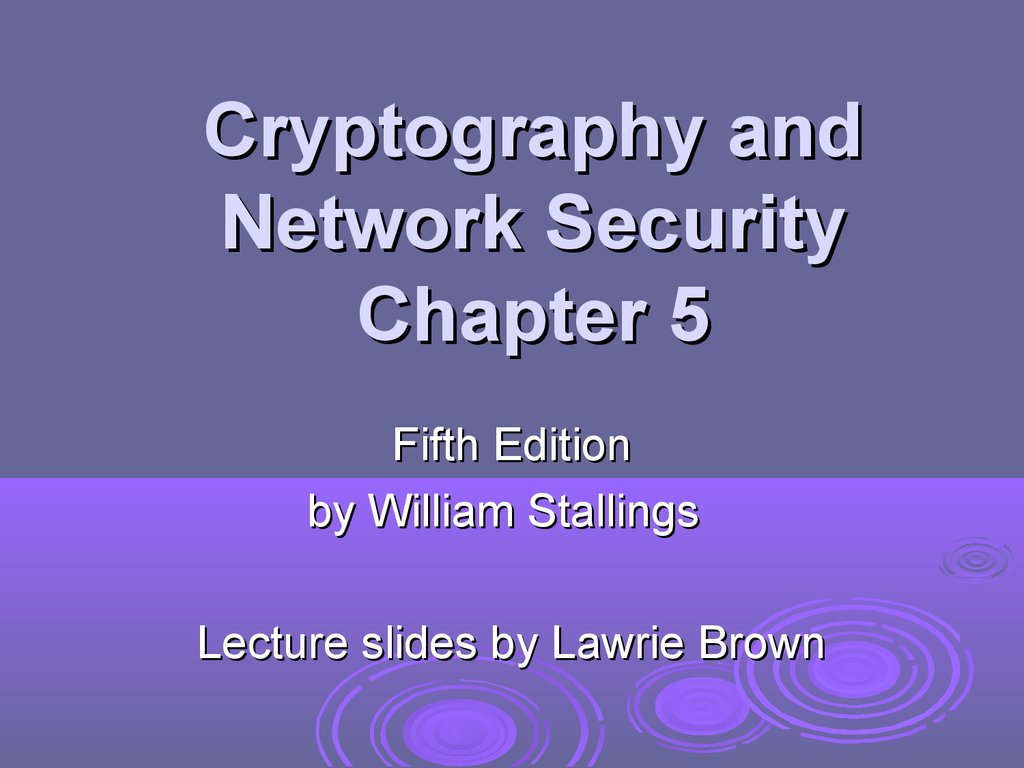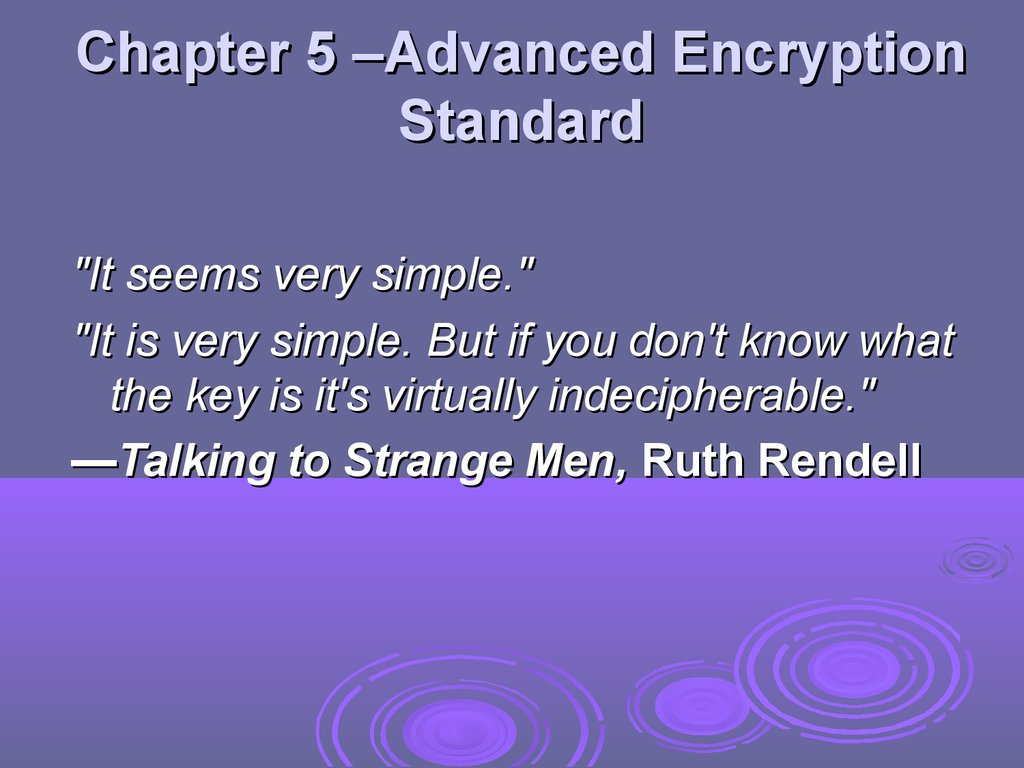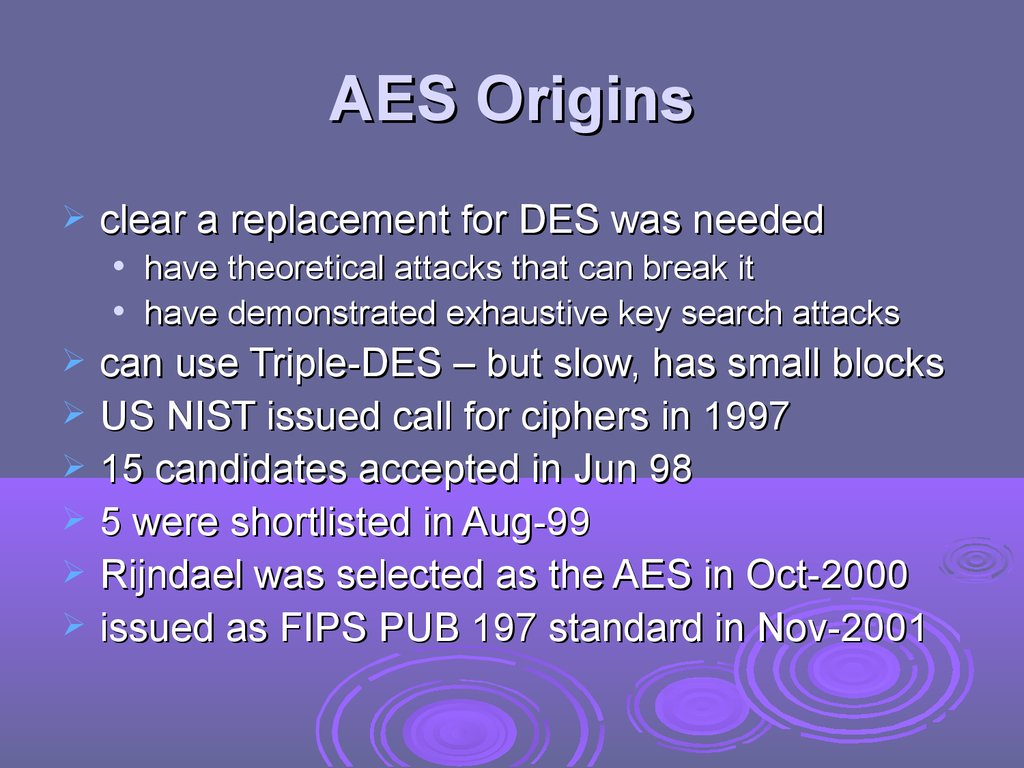# Cryptography and Network Security. Chapter 5. Fifth Edition by William Stallings

## 1.

Cryptography and
Network Security
Chapter 5
Fifth Edition
by William Stallings
Lecture slides by Lawrie Brown

## 2.

Chapter 5 –Advanced Encryption
Standard
"It seems very simple."
"It is very simple. But if you don't know what
the key is it's virtually indecipherable."
—Talking to Strange Men, Ruth Rendell

## 3.

AES Origins
clear a replacement for DES was needed
have theoretical attacks that can break it
have demonstrated exhaustive key search attacks
can use Triple-DES – but slow, has small blocks
US NIST issued call for ciphers in 1997
15 candidates accepted in Jun 98
5 were shortlisted in Aug-99
Rijndael was selected as the AES in Oct-2000
issued as FIPS PUB 197 standard in Nov-2001

## 4.

The AES Cipher - Rijndael
designed by Rijmen-Daemen in Belgium
has 128/192/256 bit keys, 128 bit data
an iterative rather than Feistel cipher
processes data as block of 4 columns of 4 bytes
operates on entire data block in every round
designed to have:
resistance against known attacks
speed and code compactness on many CPUs
design simplicity

AES
Encryption
Process

## 6.

AES Structure
data block of 4 columns of 4 bytes is state
key is expanded to array of words
has 9/11/13 rounds in which state undergoes:
byte substitution (1 S-box used on every byte)
shift rows (permute bytes between groups/columns)
mix columns (subs using matrix multiply of groups)
add round key (XOR state with key material)
view as alternating XOR key & scramble data bytes
initial XOR key material & incomplete last round
with fast XOR & table lookup implementation

AES Structure

## 8.

Some Comments on AES
1.
2.
an iterative rather than Feistel cipher
key expanded into array of 32-bit words
1.
four words form round key in each round
4 different stages are used as shown
4. has a simple structure
5. only AddRoundKey uses key
6. AddRoundKey a form of Vernam cipher
7. each stage is easily reversible
8. decryption uses keys in reverse order
9. decryption does recover plaintext
10. final round has only 3 stages
3.

## 9.

Substitute Bytes
a simple substitution of each byte
uses one table of 16x16 bytes containing a
permutation of all 256 8-bit values
each byte of state is replaced by byte indexed by
row (left 4-bits) & column (right 4-bits)
eg. byte {95} is replaced by byte in row 9 column 5
which has value {2A}
S-box constructed using defined transformation
of values in GF(28)
designed to be resistant to all known attacks

Substitute Bytes

## 11.

Substitute Bytes Example

## 12.

Shift Rows
a circular byte shift in each each
1st row is unchanged
2nd row does 1 byte circular shift to left
3rd row does 2 byte circular shift to left
4th row does 3 byte circular shift to left
decrypt inverts using shifts to right
since state is processed by columns, this step
permutes bytes between the columns

Shift Rows

## 14.

Mix Columns
each column is processed separately
each byte is replaced by a value
dependent on all 4 bytes in the column
effectively a matrix multiplication in GF(2 8)
using prime poly m(x) =x8+x4+x3+x+1

Mix Columns

## 16.

Mix Columns Example

## 17.

AES Arithmetic
uses arithmetic in the finite field GF(2 8)
with irreducible polynomial
m(x) = x8 + x4 + x3
which is (100011011)
+ x + 1
or {11b}
e.g.
{02} • {87} mod {11b} = (1 0000 1110) mod {11b}
= (1 0000 1110) xor (1 0001 1011) = (0001 0101)

## 18.

Mix Columns
can express each col as 4 equations
decryption requires use of inverse matrix
to derive each new byte in col
with larger coefficients, hence a little harder
have an alternate characterisation
each column a 4-term polynomial
with coefficients in GF(28)
and polynomials multiplied modulo (x4+1)
coefficients based on linear code with
maximal distance between codewords

## 19.

Add Round Key
XOR state with 128-bits of the round key
again processed by column (though
effectively a series of byte operations)
inverse for decryption identical
since XOR own inverse, with reversed keys
designed to be as simple as possible
a form of Vernam cipher on expanded key
requires other stages for complexity / security

Add Round Key

AES Round

## 22.

AES Key Expansion
takes 128-bit (16-byte) key and expands
into array of 44/52/60 32-bit words
start by copying key into first 4 words
then loop creating words that depend on
values in previous & 4 places back
in 3 of 4 cases just XOR these together
1st word in 4 has rotate + S-box + XOR round
constant on previous, before XOR 4th back

## 23.

AES Key Expansion

## 24.

Key Expansion Rationale
designed to resist known attacks
design criteria included
knowing part key insufficient to find many more
invertible transformation
fast on wide range of CPU’s
use round constants to break symmetry
diffuse key bits into round keys
enough non-linearity to hinder analysis
simplicity of description

AES
Example
Key
Expansion

AES
Example
Encryption

AES
Example
Avalanche

## 28.

AES Decryption
AES decryption is not identical to
encryption since steps done in reverse
but can define an equivalent inverse
cipher with steps as for encryption
but using inverses of each step
with a different key schedule
works since result is unchanged when
swap byte substitution & shift rows
swap mix columns & add (tweaked) round key

AES Decryption

## 30.

Implementation Aspects
can efficiently implement on 8-bit CPU
byte substitution works on bytes using a table
of 256 entries
shift rows is simple byte shift
add round key works on byte XOR’s
mix columns requires matrix multiply in GF(28)
which works on byte values, can be simplified
to use table lookups & byte XOR’s

## 31.

Implementation Aspects
can efficiently implement on 32-bit CPU
redefine steps to use 32-bit words
can precompute 4 tables of 256-words
then each column in each round can be
computed using 4 table lookups + 4 XORs
at a cost of 4Kb to store tables
designers believe this very efficient
implementation was a key factor in its
selection as the AES cipher

## 32.

Summary
have considered:
the AES selection process
the details of Rijndael – the AES cipher
looked at the steps in each round
the key expansion
implementation aspects